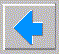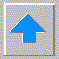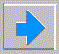# Lecture 8 - more PROLOG

• the cut
• succeeds immediately but is never resatisfied
• as side effect - commits to all before it
• so cut stops backtracking
• genesis family example
• ```    father(abraham,ishmael).
father(abraham,isaac).
is_father(X) :- father(X,_).
?-is_father(X).
abraham ; abraham
```
• in order to get the result only once ...
• ```    father(abraham,ishmael).
father(abraham,isaac).
is_father :- father(X,_), !.
?-is_father(X).
abraham
```
• the cut is like a fence
• foo :- a, b, c, !, d, e, f.
• a, b, c backtrack, but once c succeeds we never try a or b or c again
• d, e, f can later backtrack
• use of !, fail.
• ```       average_taxpayer(X) :- non_citizen(X), fail.
average_taxpayer(X) :- income<100000.
```
• this is wrong since may resatisfy later !
• so use ...
• ```       average_taxpayer(X) :- non_citizen(X), !, fail.
average_taxpayer(X) :- income<100000.
```
• let's sum from 1 to N (assume N>=1)
• ```       sum(1,1) :- !.
sum(N,R) :- N1 is N-1, sum(N1,R), R is R+N.
```
• need the cut since if had
• go :- sum(1,X), foo(Y).
• and foo(Y) fails, PROLOG will try to reinstantiate sum(1,X).
• can replace cut with use of not( ) predicate
• sum(1,1). sum(N,R) :- not(N=<1), N1 is N-1, sum(N1,R), R is R+N.
• PROLOG lists
• LISP language has list as its only data structure
• which is why it is a list processing language
• PROLOG has list a one possible data structure
• a list is a set with order
• by placing lists inside lists we can make tree structures
• examples:
• [ ] the empty list
• [a, b, c, d] a list with four elements
• [a, [b,c], d] a list with three elements, the second of which is a list
• one manipulation of lists is to separate head from tail
• head is the first elements in the list, the tail is the rest of the list (a list!)
• [ head | tail ]
• there are potential problems with this notation, e.g. [A|B] = [X,Y]
• ?- [H, T] = [a, b, c, d]. H=a T=[b,c,d]
• ?- [H,T] = [a]. H=a, T=[ ]
• ?- [H,T] = [ ]. no
• dot notation - binary tree interpretation
• [a] == .(a,[])
• [a,b,c] == .(a,.(b,.(c,[])))
• some LISP-like predicates (most with 2 uninstantiated variables)
• append
• ?- append([a,b,c], [1,2,3], [a,b,c,1,2,3]). yes
• ?- append([a,b], [c,d], X). X=[a,b,c,d]
• ?- append(X, [b,c,d], [a,b,c,d]). X=[a]
• how is append defined ?
• ```        append([ ], L, L).
append([H | L1], L2, [H | L3]) :- append(L1, L2, L3).
```
• is_list
• tells if instantiated variable is a list
• note that not all [A|B] are lists - only if B is a list !
• how is is_list defined ?
• ```        is_list([]).
is_list( [A | B] ) :- is_list(B).
```
• meaningless for uninstantiated variable
• 1) use var predicate
• 2) use weaker test, e.g. is_list([]). is_list([_,_]). (but some non-lists will pass)
• member
• defined in the following way:
• member(X, [X | _ ]).
• this is better than defining
• member(X, [Y | _ ] :- X=Y.
• member(X, [_ | Y]) :- member(X,Y).
• last
• ```      last(X,[X]).
last(X,[_|Y) :- last(X,Y).
```
• append can often be used instead
• last(L,List) := append(_,[L],List).
• member(M,List) :- append(_,[M|_], List).
• recursion
• basic tools in AI languages such as PROLOG and LISP
• need to ensure termination (easy for lists and the cut)
• first example
• is_integer: is_integer(0).
• is_integer(X) :- is_integer(Y), X is Y+1.
• answers are 0; 1; 2; 3 ...
• classic exmaple of recursion is factorial
• ```     factorial(1,1).
factorial(N,R) :- N>1, N1 is N-1, factorial(N1,Tmp), R is N*Tmp.
```
• need N>1 to terminate the recursion
• more elegant with cut
• ```     factorial(1,1) :- !.
factorial(N,R) :- N1 is N-1, factorial(N1,Tmp), R is N*Tmp.
```
• Hanoi rings puzzle
• ```       hanoi(N) :- move(N,left,center,right).
move(0,_,_,_) :- !.
move(N,A,B,C) :- M is N-1, move(M,A,C,B), print(‘move a disc from ‘, A, ‘ to ‘, B), nl, move(M,C,B,A).
```
• recursion is often used with lists
• ```    printlist([]).
printlist([H|T]) :- print(H),printlist(T).
```
• infinite recursion
• ```       parent(X,Y) :- child(Y,X).
child(A,B) :- parent(B,A).
```
• left recursion (fix by reversing order in rule)
• ```        person(X) :- person(Y), parent(Y,X).
```
• some particularly useful recursions
• repeat
• defined as repeat :- repeat.
• e.g. get0(C) :- repeat, get(X), X>32, !.
• Wumpus world in Prolog (agent part, need simulator also)
• ```   can_enter(X,Y) :- not_wumpus(X,Y), not_pit(X,Y).
not_wumpus(X,Y) :- not(stench(X1,Y1)), adjacent(X,Y,X1,Y1).   and same for not_pit
adjacent(X,Y,X1,Y1) :- X1is X-1, Y1 is Y.
adjacent(X,Y,X1,Y1) :- X1 is X+1, Y1 is Y.  etc.
pickup_gold(X,Y) :- glitter(X,Y).
climb_out(X,Y) :- X=1, Y=1, have_gold.
forward(X,Y) :- angle=0, X is X=1, update(X,Y).  update is simulator function
```